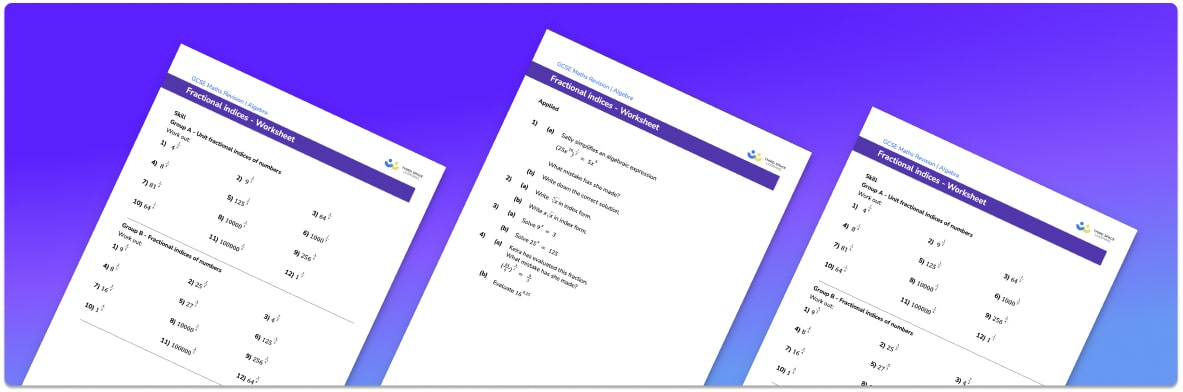# Fractional Indices Worksheet• Section 1 of the fractional indices worksheet contains 20+ skills-based fractional indices practice questions, in 3 groups to support differentiation
• Section 2 contains 3 applied fractional indices questions with a mix of worded problems and deeper problem solving questions
• Section 3 contains 3 foundation and higher level GCSE exam questions on fractional indices
• Answers and a mark scheme for all fractional indices questions are provided
• Questions follow variation theory with plenty of opportunities for students to work independently at their own level
• All questions created by fully qualified expert secondary maths teachers
• Suitable for GCSE maths revision for AQA, OCR and Edexcel exam boards

• This field is for validation purposes and should be left unchanged.

You can unsubscribe at any time (each email we send will contain an easy way to unsubscribe). To find out more about how we use your data, see our privacy policy.

### Fractional indices at a glance

Fractional indices are exponents which are fractions. The denominator of the fraction tells us to take a specific root of the base number (such as the square root or the cube root etc.), whilst the numerator tells us the power to raise this to. For example, let’s look at 16 raised to the power of 3 over 2. The denominator of the power is 2 so we take the square root of 16 which is 4. The numerator of the power is 3 so we raise 4 to the power of 3 to give 64.

When we have fractional or negative indices, the rules of indices still apply (e.g. adding the powers when multiplying terms, subtracting the powers when dividing terms).

We could also have negative fractional indices which tell us to take the reciprocal.

Looking forward, students can progress with more indices worksheets to additional algebra worksheets for example a simplifying expressions worksheet or simultaneous equations worksheet.For more teaching and learning support on Algebra our GCSE maths lessons provide step by step support for all GCSE maths concepts.

## Do you have KS4 students who need more focused attention to succeed at GCSE?There will be students in your class who require individual attention to help them succeed in their maths GCSEs. In a class of 30, it’s not always easy to provide.

Help your students feel confident with exam-style questions and the strategies they’ll need to answer them correctly with our dedicated GCSE maths revision programme.

Lessons are selected to provide support where each student needs it most, and specially-trained GCSE maths tutors adapt the pitch and pace of each lesson. This ensures a personalised revision programme that raises grades and boosts confidence.

Find out more